# Volume of Composite Solid Figures

 Listen to this Lesson:

When learning about the volume of composite solid figures, fifth graders are expected to compose and decompose figures into smaller rectangular prisms and understand how the volume of the figure is the sum of the smaller rectangular prisms.

They should also achieve fluency in using the additive property of volume to find the volume of composed figures, as well as in using the appropriate methods to solve word problems involving the volume of rectangular prisms.

Even though teaching volume can be loads of fun as it engages the visual spatial reasoning of kids, it remains one of the most misunderstood math concepts among students and one of the trickiest topics to teach them.

However, there are a couple of things that you could use in your classroom to smoothen out the learning process when teaching volume in general and volume of composite solid figures in particular. Read on and learn more.

## Ideas for Teaching Volume of Composite Solid Figures

### Review Volume

It’s a good idea to start your lesson on volume of composite solid figures by reviewing the concept of volume first. Even though this is something that students should be familiar with, it will help you identify any existing gaps in their understanding.

Define volume as a measurement of the capacity of objects. Point out that an object must have these dimensions: length, width, and height, for it to have volume. Present an example on the whiteboard of an object with the mentioned dimensions, for instance:### Volume of Rectangular Prisms

Now that students have a solid understanding of what volume is, you can proceed with explaining how to measure the volume of rectangular figures. First, remind students that rectangular prisms are three-dimensional figures made up of six faces of rectangles, such as: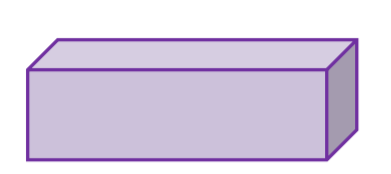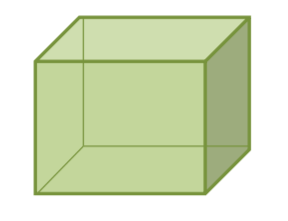Then, explain that we can find the volume of rectangular prisms by using the appropriate method depending on the given data. Point out that there are different methods to do this, including:

• when we have the unit cube’s volume or dimensions, we will count the cubes to find the volume
• when we know the base area and height, we will use the following formula: base × area × height
• when the length, width, and height are given, we will apply this formula: length × width ×height

#### Example 1:

Present an example where we have the unit cube’s volume or dimensions and illustrate how we can measure the volume of the given rectangular prism. Start by drawing the prism on the whiteboard:Then, add that one cube has a volume of 1 in3. Explain that since the rectangular prism has a total of 24 cubes, the total volume of the prism would be 24 in3.

#### Example 2:

Present an example where we know the base area and height and illustrate how we can measure the figure’s volume. Draw the rectangular prism on the whiteboard, and mark the given height and base area: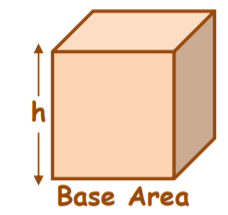Add that we know that the base area is 14 cm2 and the height is 4 cm. Point out that we will use the following formula: base area × height to measure the volume of the prism, which means that the total volume of the prism is: 14× 4 = 56 cm3.

#### Example 3:

Present an example where we know the length, width, and height, and demonstrate how we can find the volume of the given rectangular prism. Draw the prism on the whiteboard and mark the length, width, and height in the following way: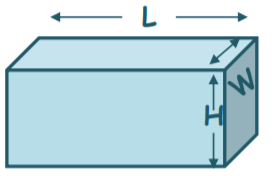Add that we know that the length is 10 ft, width is 5 ft, and height is 15 ft. Point out that we will use this formula: length × width × height to measure the volume of the prism, which will result in 10 × 5 × 15 = 750 ft3.

### Composite Figures

Explain to students that composite figures (also known as composed figures) are figures made up of two or more simpler solid figures. We’ll be learning about composed figures made up of rectangular prisms in this section.

Present a few drawings of such composite figures on the whiteboard. Point out that we can decompose each of these composite figures to show how they are made up of different rectangular prisms:Present examples of we can decompose such figures, for instance:#### Volume of Composite Solid Figures

Now that students have learned what composite figures represent and how we can decompose them into smaller rectangular prisms, you can move on to demonstrating how we can apply this understanding when measuring the volume of composite solid figures.

Explain that to achieve this, we can simply add up the volume of all the rectangular prisms that compose a composite figure. That is, we can decompose any given composed figure to show how they are made up of different rectangular prisms and then add up the separate volumes.

##### Example:

Draw two rectangular prisms on the whiteboard. Color one prism orange and the other one purple. Point out that the orange rectangular prism has a volume of 24 cm3 and the purple rectangular prism has a volume of 18 cm3.

So the volume of the composed figure would be 18 cm3 + 24 cm3 = 42 cm3: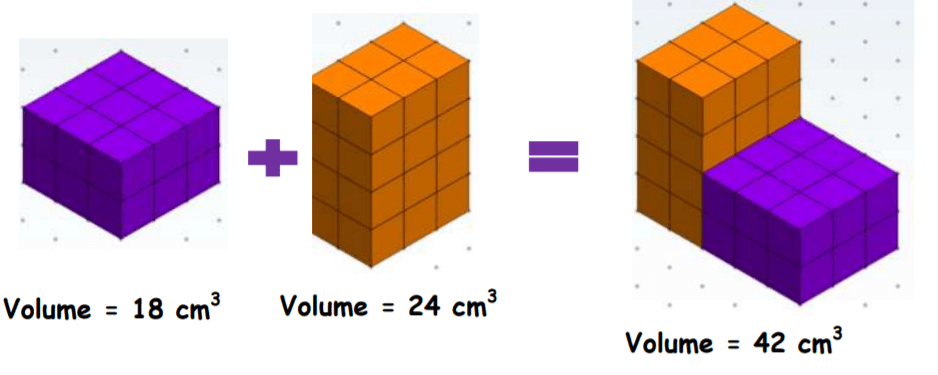Explain that if the volumes of the rectangular prisms are not given yet, we will apply the methods we’ve learned in finding the volume of a rectangular prism. Let’s say we have a composite figure with these dimensions and values given: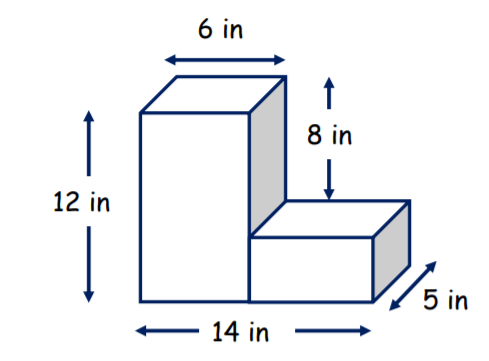Point out that first, we’ll decompose the given composite figure, and find the individual volumes of the rectangular prisms. Then, we will add up the individual volumes: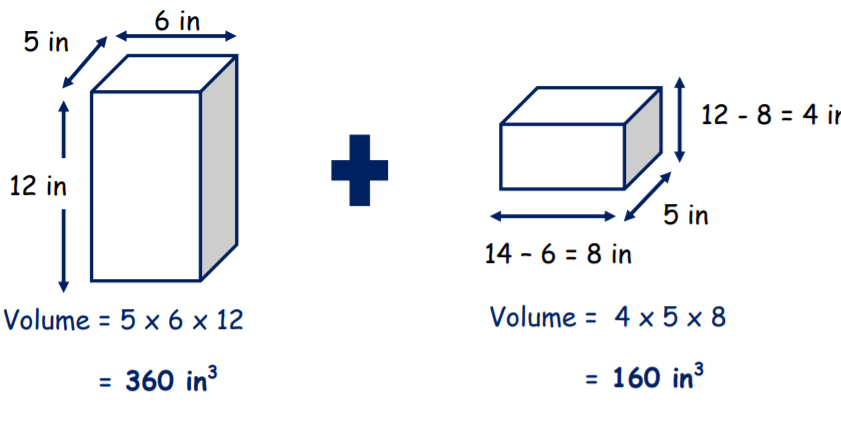Explain that in this case, the length, width, and height are given, which means that we will apply this formula: length × width × height to find the total volume. By doing so, we see that the individual volumes are 360 in3 and 160 in3. Adding these up results in a total volume of 520 in3.

If you have the technical possibilities in your classroom, you can also enhance your lesson on the volume of composite solid figures with multimedia materials, like videos for example. Videos will come in handy for better visualizing the process of decomposing the given figures.

For starters, play this video as an introduction to the concept of volume to brush up on students’ knowledge of volume. Then, show this video to provide an introduction to measuring the volume of rectangular prisms with unit cubes.

This video is a great resource on measuring the volume of a rectangular prism when the base area and height are given, whereas this one is good for explaining how to measure the volume of a rectangular prism when the length, width, and height are given.

Finally, use this video to illustrate to students how to find the volume of composite solid figures. The video demonstrates how by decomposing the figure into two rectangular prisms, finding their volumes, and then adding them up, we can find the volume of the composite figure.

## Activities to Practice Volume of Composite Solid Figures

### Volume of Irregular Figures

This is a simple, online game that you can use for reviewing students’ understanding of measuring the volume of irregular figures made of unit cubes. To implement this game in your class, just make sure that there is a suitable device for each student.

Students play the game individually, making it suitable for homeschooling parents as well. Remind students that when we have the unit cube’s volume or dimensions, we will count the cubes to find the volume. Provide a few minutes to complete the exercises then discuss them.

### Decompose Figures to Find Volume

In this online game, students get to practice finding the volume of composite figures. In order to use the game in your class, the only thing you’ll need are suitable technical devices for all students.

Students play the game individually. In each exercise, students have to find the volume of a given figure, by composing and decomposing figures into smaller rectangular prisms, using their understanding of how the volume of the figure is the sum of the smaller rectangular prisms.

Once students finish the game, allow time for discussion and reflection. To how many prisms did the students decompose the given composite figures? How did they find the volumes of each prism and composite figure?

### Pair Work

This activity offers the opportunity to hone students’ knowledge of how to find the volume of composite figures. To use this activity in your class, you’ll need to print out this Assignment Worksheet (Members Only).

Divide students into pairs and hand out the worksheets (one copy per student). Provide instructions and add that students in each pair work together to solve the exercises in the worksheets. In each exercise, students are asked to find the volume of composite figures.

By decomposing them into smaller rectangular prisms, students should use the appropriate method to find the volume of each prism and then add them up to calculate the volume of the composite figure. Before starting the game, you can write the methods on the whiteboard.

Point out that in cases where we know the base area and height, students should use the appropriate formula of base area × height, and in cases where the length, width, and height are given, they should use this formula: length × width × height.

Provide a few minutes for students to complete the worksheets. Then, give them time for discussion and reflection. Which exercises were more challenging? Which method did students use to find the volume of the given figure? Why?

## Before You Leave…

If you enjoyed these ideas and activities for teaching how to find the volume of composite solid figures, you may want to check out our lesson that’s dedicated to this topic!